The problem of the geometric characterization of domains in a given analytic space that are Stein spaces (cf. Stein space); it was posed by E.E. Levi  for domains in the affine spacein the following form. Letbe a domain ineach boundary pointof which has the following property: there is a neighbourhoodof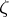inand a holomorphic function inthat cannot be extended holomorphically to. Isa domain of holomorphy? This property is equivalent to any of the following assertions about the domain: 1) for no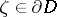is there a sequence of bounded holomorphic surfaces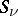that converges to a holomorphic surfacewith,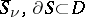,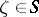; 2) the domainis pseudo-convex, that is,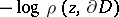,, whereis the Euclidean distance, is a plurisubharmonic function in; and 3)is a pseudo-convex manifold, that is, there is ina plurisubharmonic function that tends toas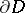is approached. The Levi problem forwas affirmatively solved in 1953–1954 independently by K. Oka, H. Bremermann and F. Norguet, and Oka solved the problem in a more general formulation, concerned with domains spread over(cf. Covering domain) (see –). Oka's result has been generalized to domains spread over any Stein manifold: If such a domainis a pseudo-convex manifold, thenis a Stein manifold. The Levi problem has also been affirmatively solved in a number of other cases, for example, for non-compact domains spread over the projective space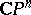or over a Kähler manifold on which there exists a strictly plurisubharmonic function (see ), and for domains in a Kähler manifold with positive holomorphic bisectional curvature . At the same time, examples of pseudo-convex manifolds and domains are known that are not Stein manifolds and not even holomorphically convex. A necessary and sufficient condition for a complex space to be a Stein space is that it is strongly pseudo-convex (see Pseudo-convex and pseudo-concave). Also, a strongly pseudo-convex domain in any complex space is holomorphically convex and is a proper modification of a Stein space (see ,  and also Modification; Proper morphism).
The Levi problem can also be posed for domainsin an infinite-dimensional complex topological vector space. Ifis locally convex andis a domain of holomorphy, thenis pseudo-convex, that is, inthere is a plurisubharmonic function that tends to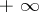asis approached. The converse theorem is false even in Banach spaces, but it has been proved for Banach spaces with a countable basis, as well as for a number of other classes of spaces(see ).